## ∂Lux

Louis Desdoigts
The University of Sydney
Slides availale

louisdesdoigts.github.io/diff_optics/Code and examples available

Work in collaboration with:
Benjamin Pope (UQ)
Peter Tuthill (USyd)
Jordan Dennis, student (UQ)
You can watch these slides being presented here!

## Inference & Design Problems in Optics

JWST, Toliman, & Beyond!

Phase Retireval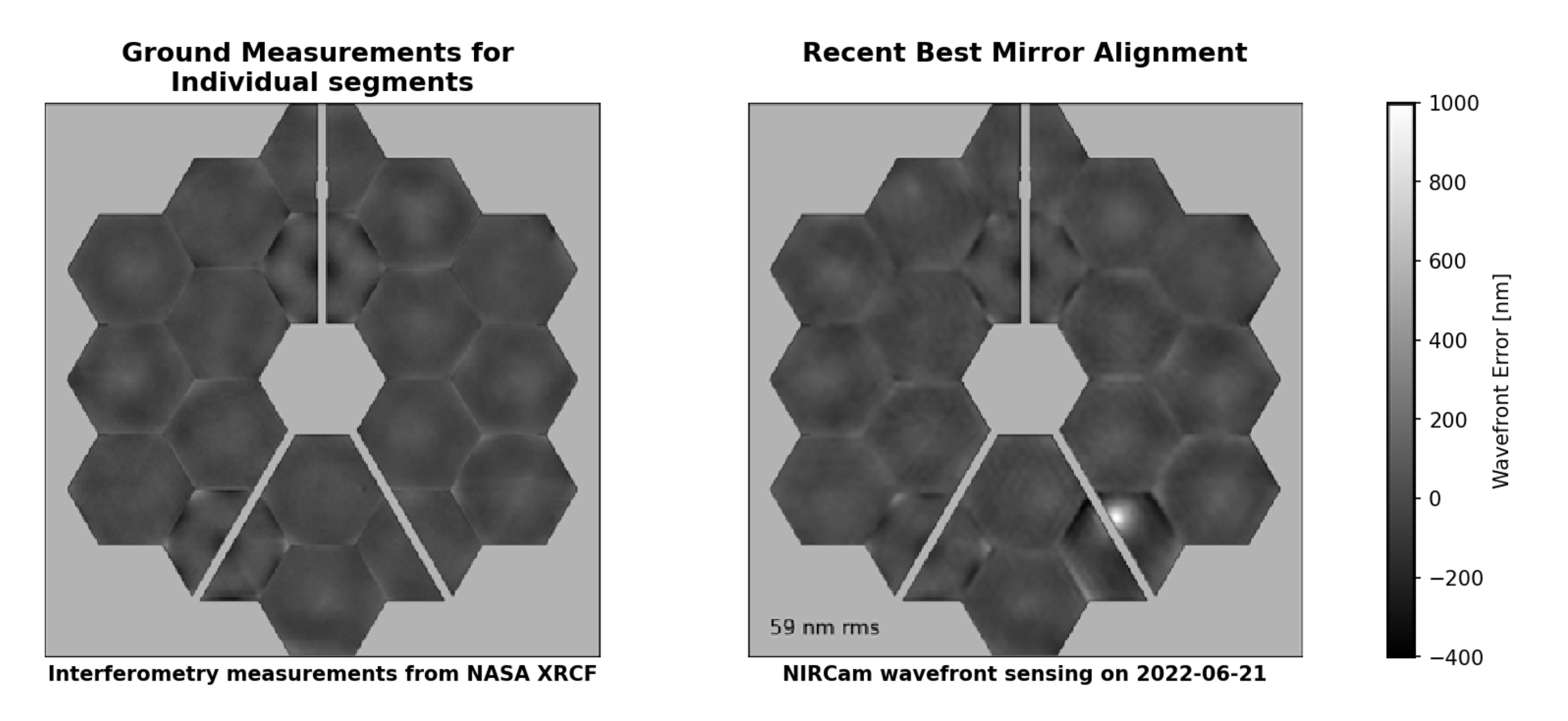Instrumental Calibration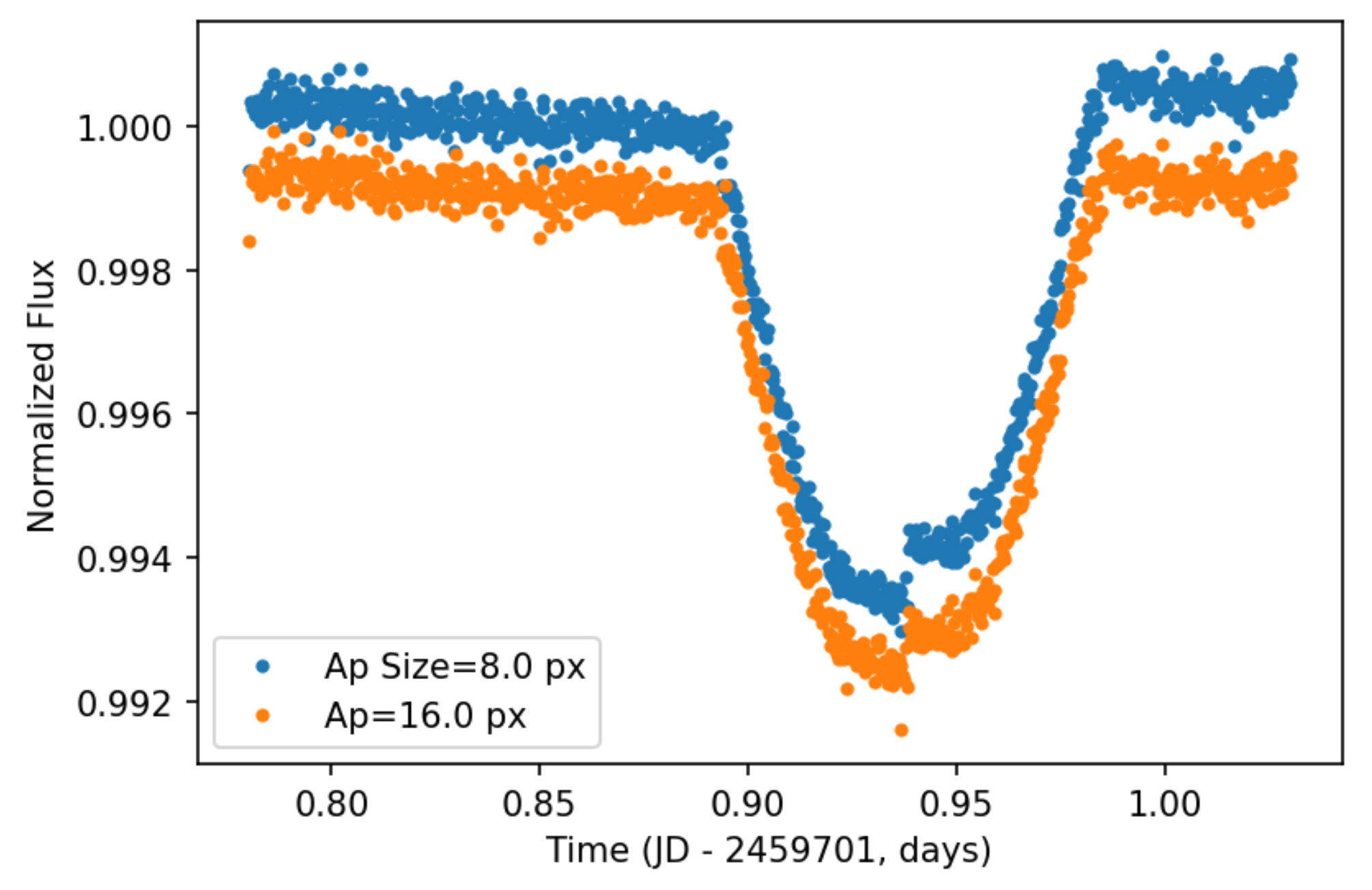### Toliman

μ-arcsecond astrometry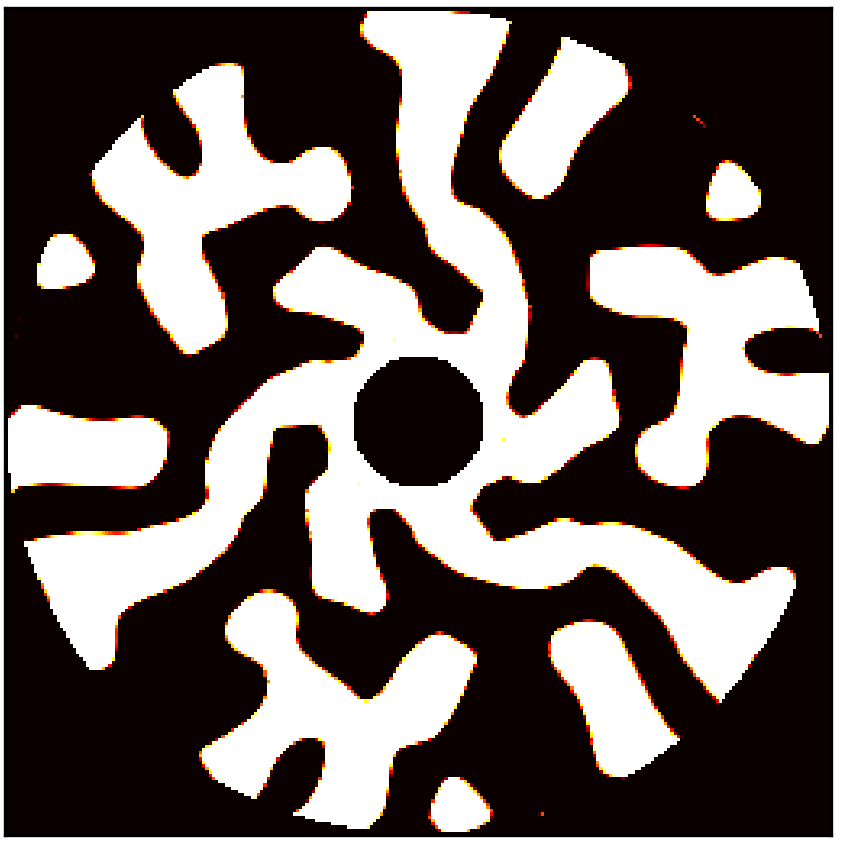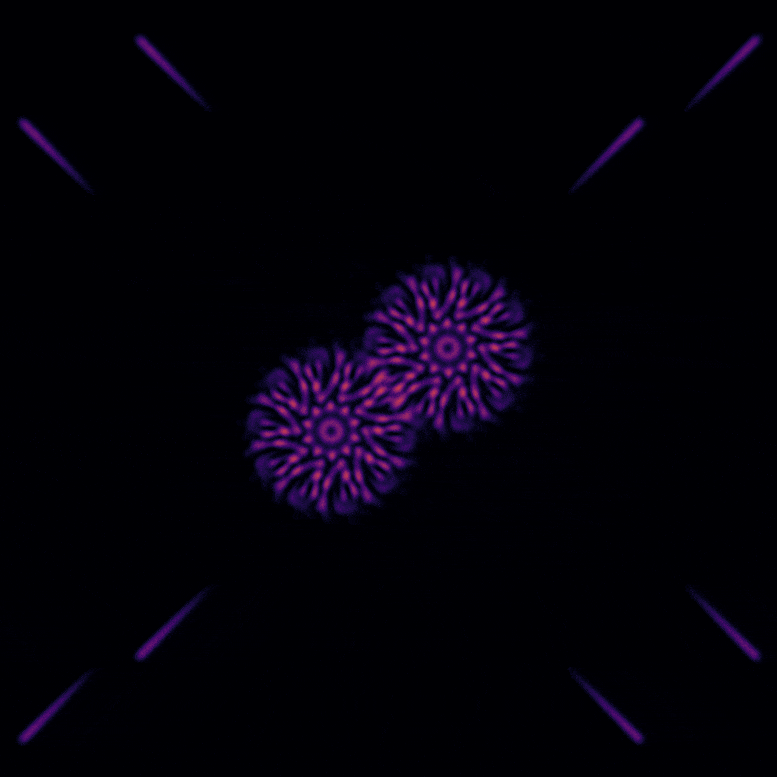### Beyond!

Low-order wavefront error resistant coronagraphs

Polarisation invariant coatings

Non-common path error correction in AO

Crowded-field signal decontamination

## Automatic Differentiation & Optics

Unifying Machine Learning and Optics

### Isomorphism

What does a Neural Network DO?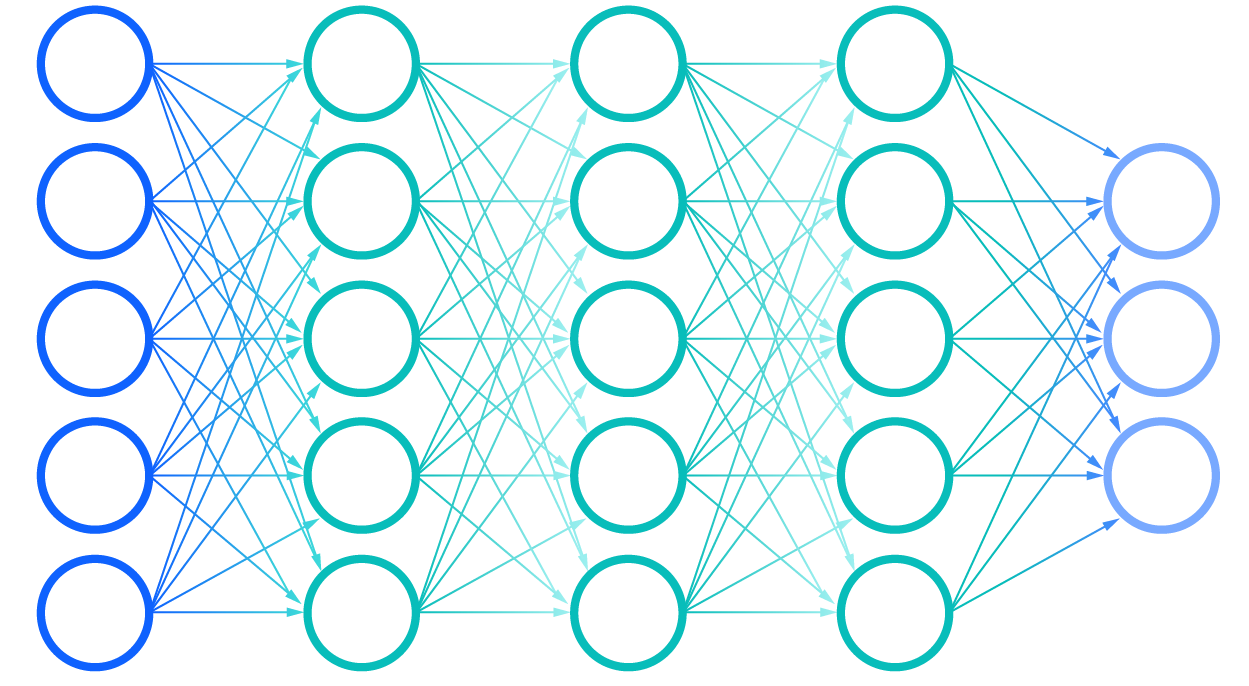### Isomorphism

What does an Optical System DO?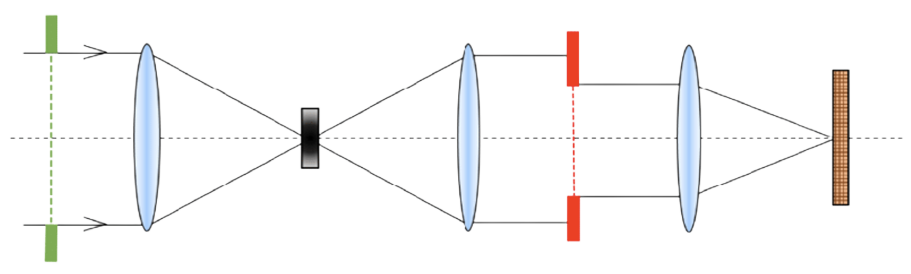Neural Network and Optical Systems are structurally isomorphic

Optical forwards models constructed within ML frameworks can harness Automatic Differentiation!

### Automatic Differentiation

What is Autodiff?

• It is NOT finite differencing
• It is NOT symbolic differentiation
• It IS repeated applications of the chain rule to code in order to calcualte derivates

### Automatic Differentiation

Why is autodiff usefull?

• Computational cost of derivative calcualtions do Not scale with the number of parameters
• Complex, high-dimensional models can optimised simply
• Parameter gradients let us access a host of better optimsation and inference algorithms!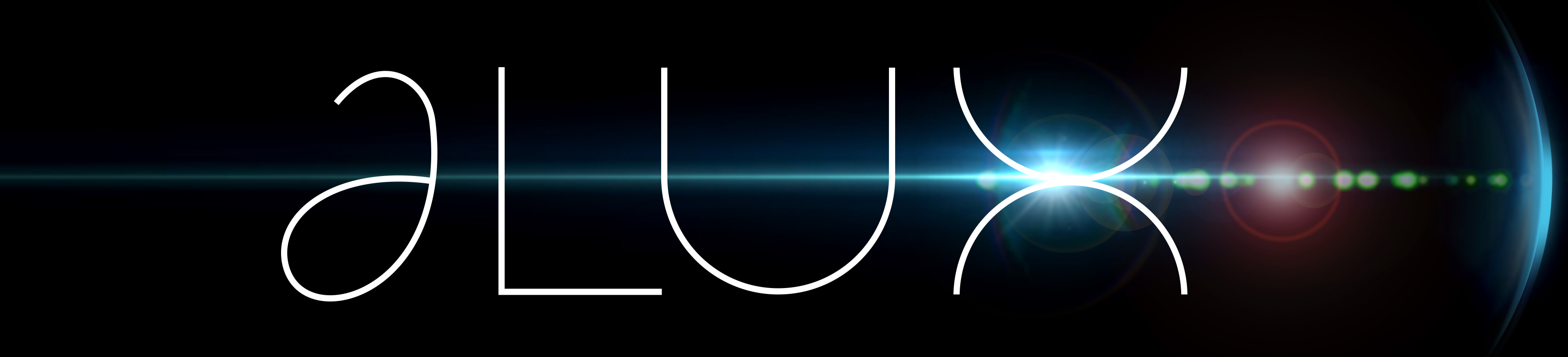∂Lux - An open source, Python based, fully differentiable optical modelling framework using Google Jax & Equinox

## ∂Lux

• Automatic Differentiation!
• Just-in-time (jit) compilation
• Accelerated Linear Algebra (XLA) - Optimised for GPUs!
• Object-Oriented models
• Integration with ML optimisation libraries (Optax)
• Integration with PPLs (Numpyro)

Tutorial

(CLIMB)

## CLIMB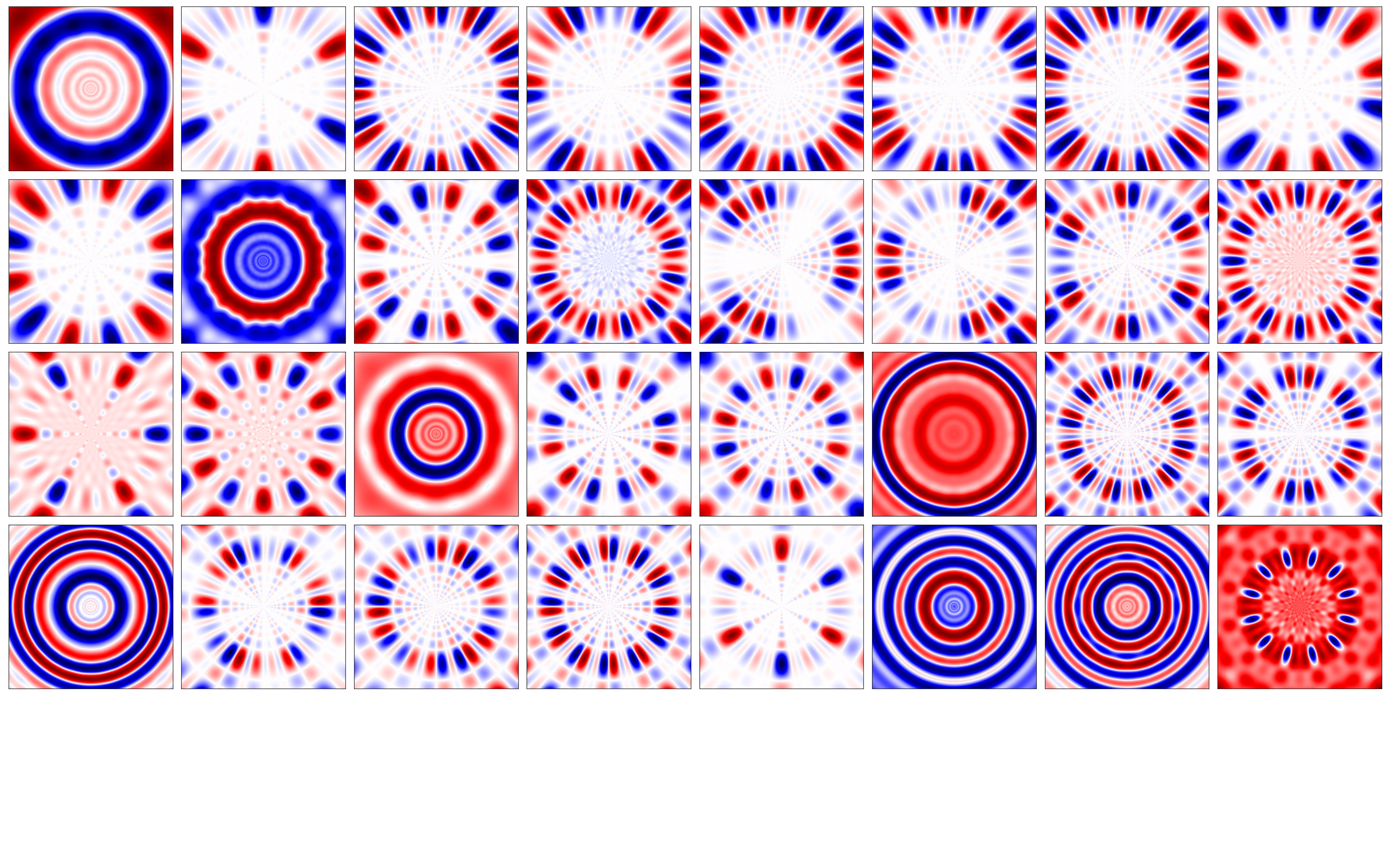## CLIMB

How do you design a mask to best constrain binary-star separation AND the instantaneous state of the telescope?

Engineer a loss function!

$\text{Loss}(\psi, p) = - \sum_{(x, y)} \Big(\nabla \psi(x, y) (\alpha + \beta\vec{r})\Big)^p$

## Optical Design

Tutorial

How do we compare performace?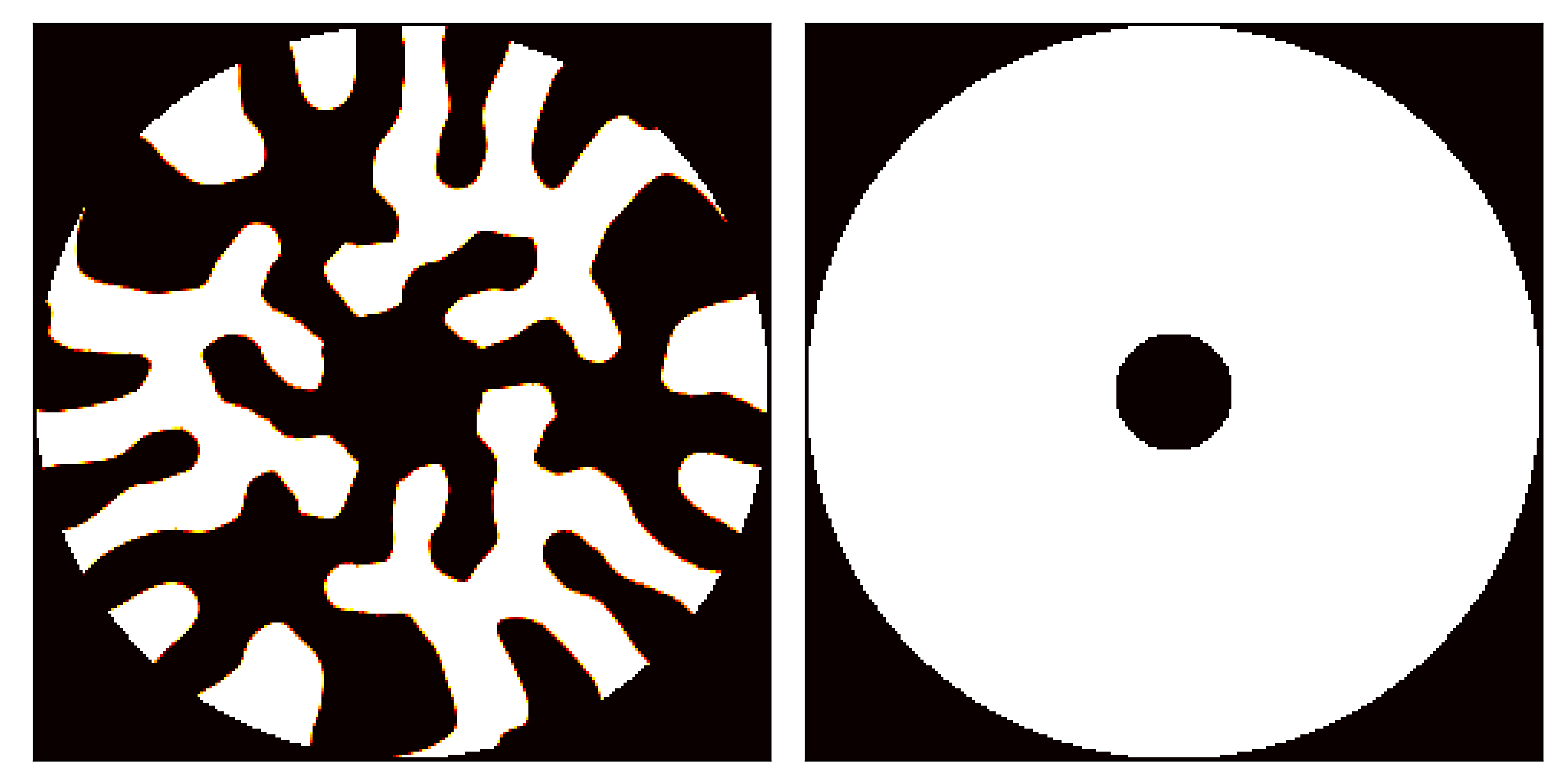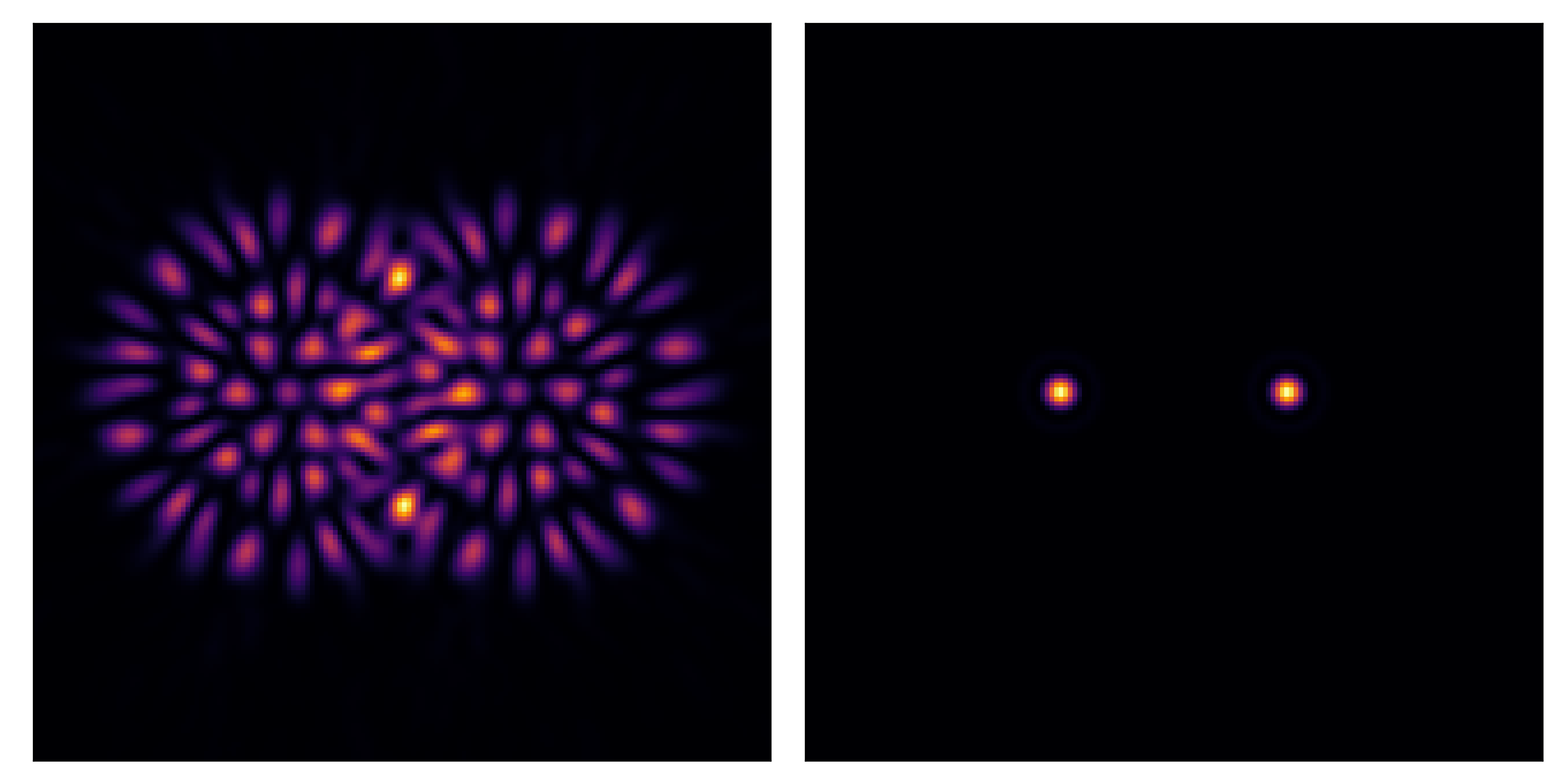Cramer-Rao lower-bound calcualtion under the Laplace approximation

$\text{CRLB}(\vec{X}) \propto \frac{1}{\vec{\Sigma}(\vec{X})}$ where $\vec{\Sigma}(\vec{X}) = - \Big[ \nabla \nabla \text{ln} \Big( \mathcal {L}(\vec{X}) \rho(\vec{X}) \Big) \Big]^{-1}$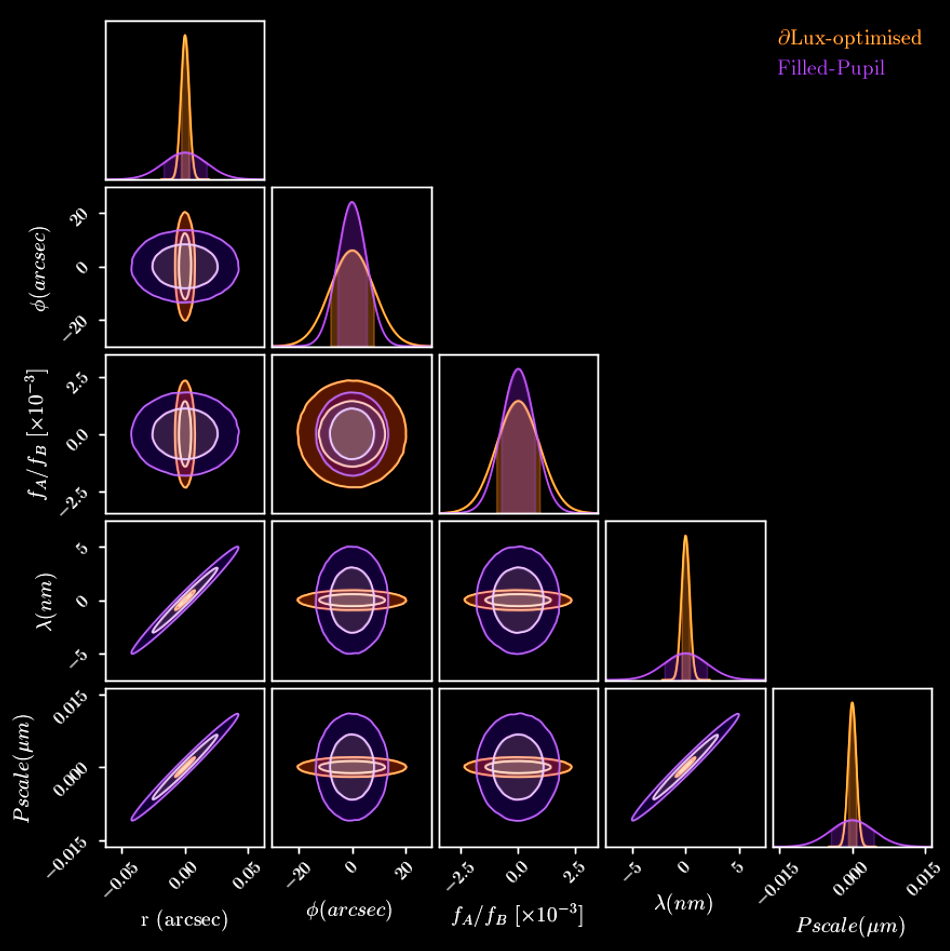Going further...

What if we optimised the entropy of the covariance matrix?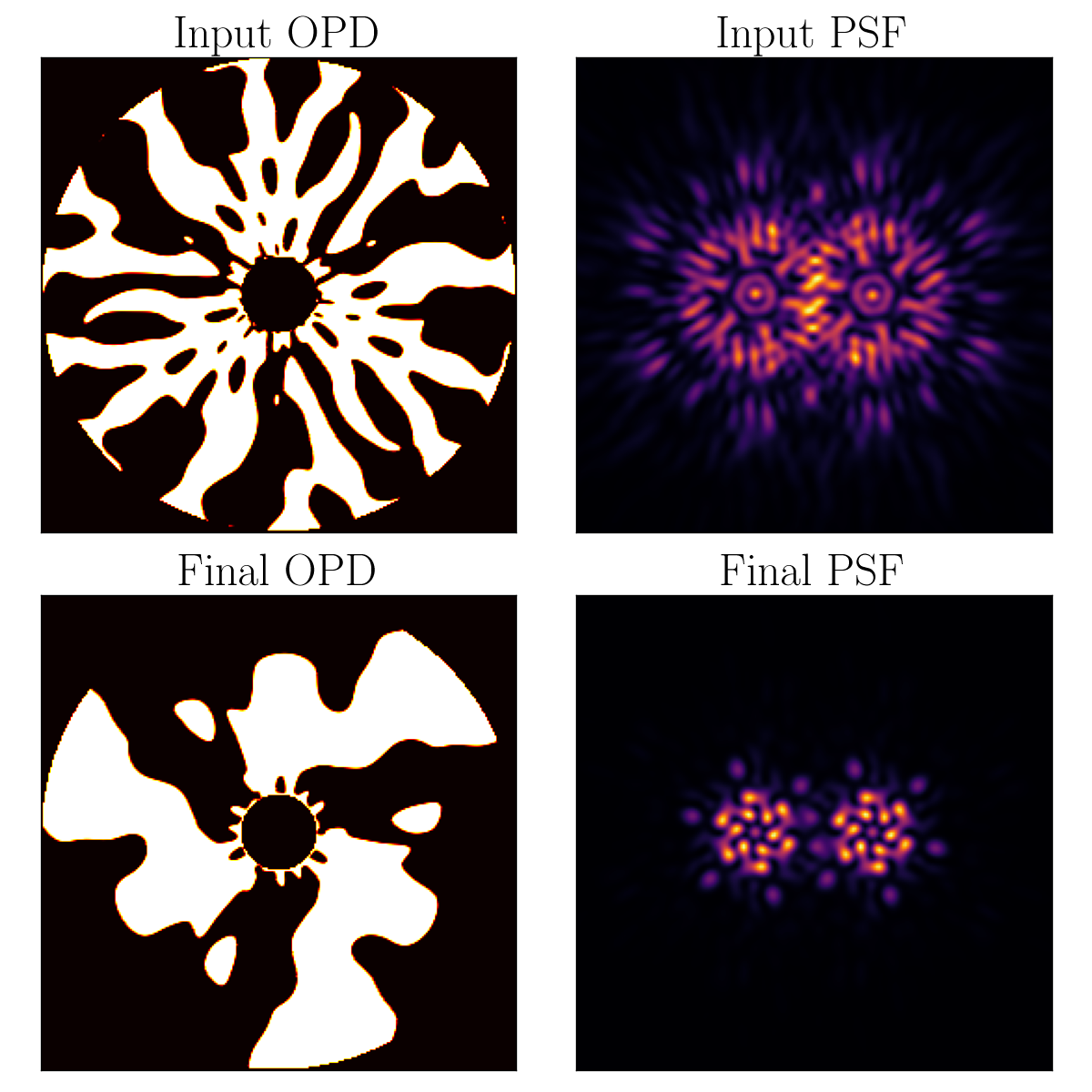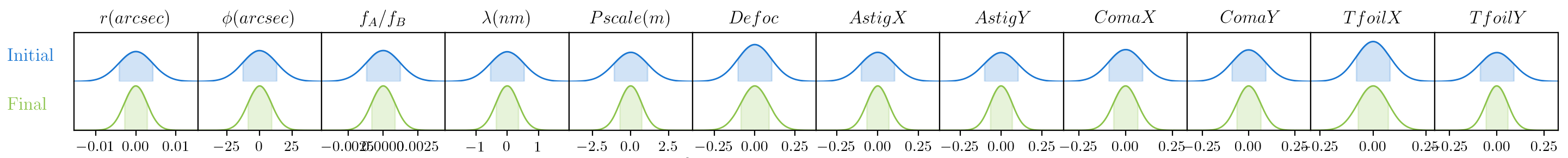## Data Analysis

Demonstration

How to understand relationships between a large number of interrelated parameters from data?

Hamiltonian Monte Carlo (HMC)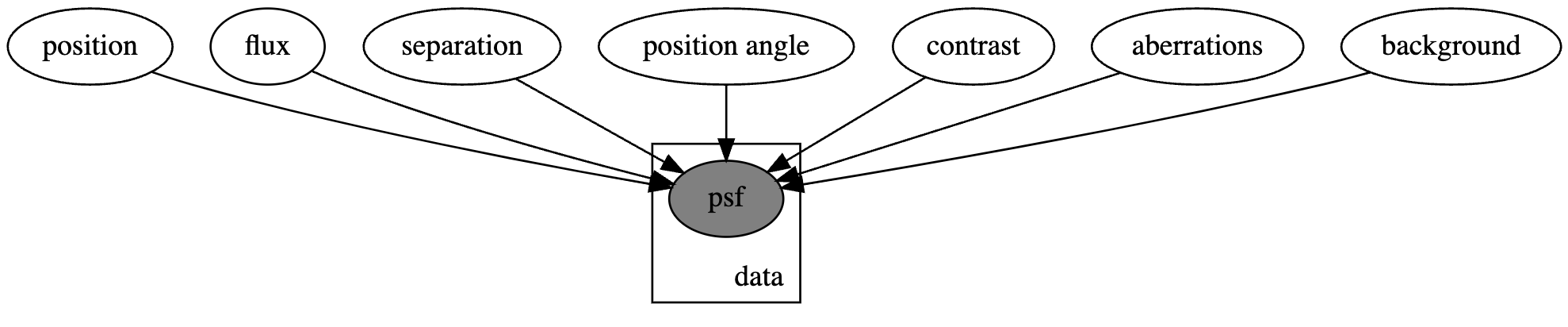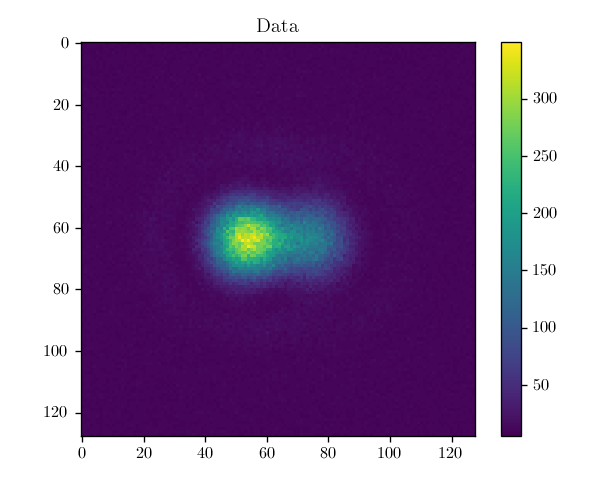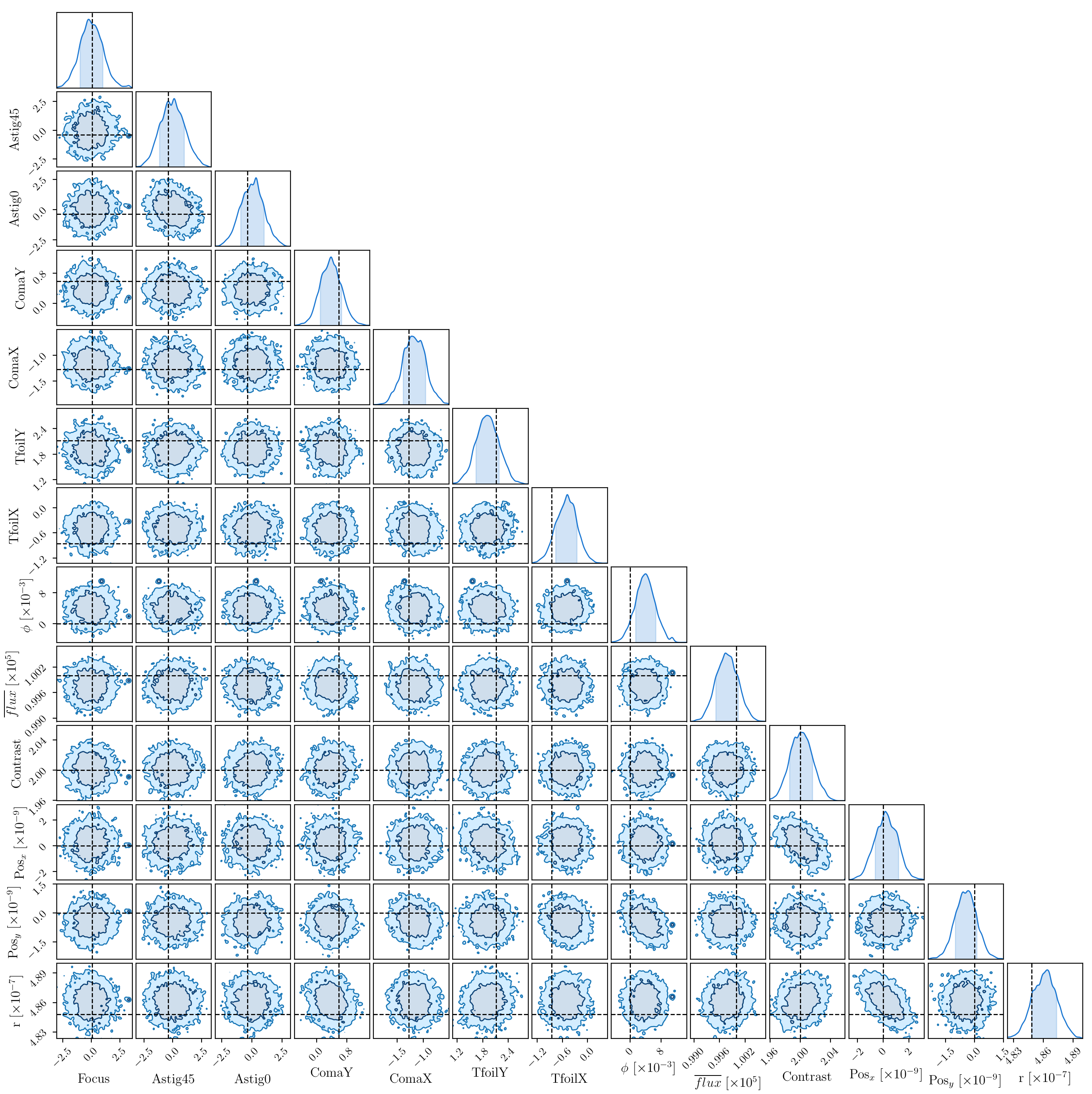## Phase Retrieval & Instrumental Calibration

Tutorial

Data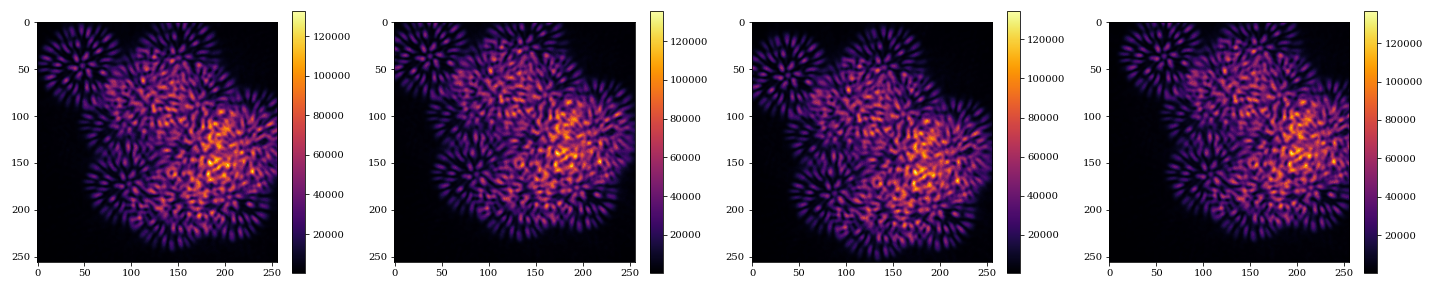Initial Resdiuals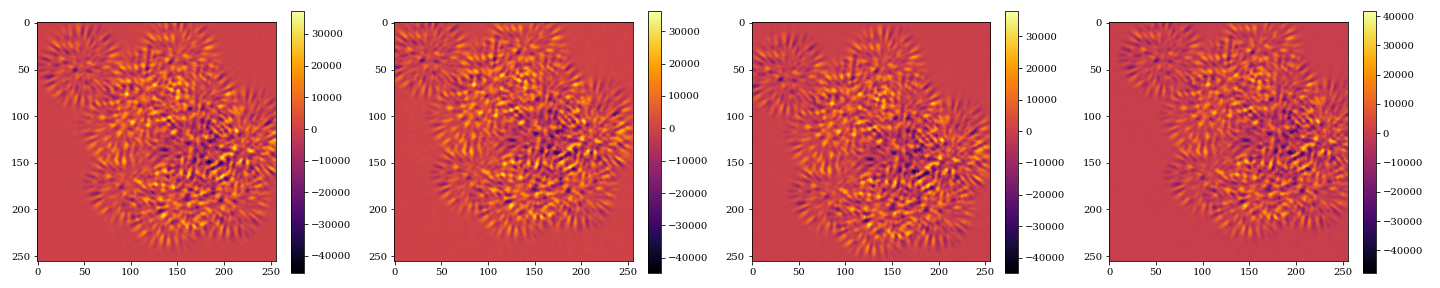• Stellar positions & fluxes (45 parameters)
• Optical aberrations (11 parameters)
• Interpixel sensitivites (65'536 parameters)

Astrophysical parameter recovery

Optical aberration recovery

Inter-pixel sensitivity recovery

## Future Work

Bulk optic design with ray tracing